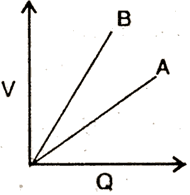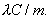Thursday 13th August 2020

CBSE Guess > Papers > Question Papers > Class XII > 2004 > Physics > Delhi Set-II

PHYSICS (Set II—Delhi)

Except for the following questions all the remaining question have been asked in Set I.

Q. 3. What is the name given to that part of electromagnetic spectrum which is used in ‘Radar’? 1

Q. 5. Which physical quantity has the unit Wh/m2? Is it a scalar or a vector quantity? 1

Q. 6. Draw a circuit diagram-to show how a photo-diode is biased. Draw its characteristic curves for two different illumination intensities. 2

Q. 7. Find the wavelength of electromagnetic waves of frequency 4 x 109 Hz in free space. Give its two applications. 2

Q. 8. Draw the graph showing the variation of binding energy per nucleon with mass number. Explain, using this graph, why heavy nuclei can undergo fission. 2

Q. 12. The graph shows the variation of voltage, ‘V’ across the plates of two capacitors A and B versus increase of charge ‘Q’ stored on them. Which of the two capacitors has lower capacitance? Give reason for your answer. 2Q. 16. Derive an expression for (i) induced e.m.f. and (ii) induced current. when a conductor of length ‘l’ is moved with a Uniform velocity normal to a uniform magnetic field B. Assume the resistance of the conductor to be ‘R’. 3

Q. 18. State Gauss’ theorem in electrostatics. Using this theorem, derive an ex pression for the electric field intensity due to an infinitely long, straight wire of linear charge density. 3

Q. 21. Write the logic symbol and truth table for OR gate. Explain how Ibis gate can be realized by using two diodes.

 Physics 2004 Question Papers Class XII Delhi Outside Delhi Compartment Delhi Compartment Outside DelhiSet ISet ISet ISet ISet IISet IISet IISet IISet IIISet III

CBSE 2004 Question Papers Class XII